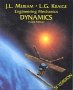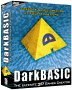# Subject: angular velocity in rotating frame-of-reference

From: "Martin Baker"
Subject: angular velocity in rotating frame-of-reference
Date: 03 December 2002 18:17

Could anyone help me derive an equation relating the angular velocity
of an solid object when viewed in a local frame-of-reference and when
viewed in an absolute frame-of-reference (when the frame-of-reference
is itself rotating - about a different point). Can I just add the
local angular velocity to the frame-of-reference angular velocity?
(even though they are rotating about different points? Or does the
angular velocity of an solid object not have any meaning when it has
this sort of motion?

Would it help if I made some assumptions (such as the object is
rotating about its own centre of mass).

I have made an attempt to solve this here, but I've got bogged down:

Thank you for any help or ideas of how to proceed.

Martin

From: "John Nagle" <nagle@animats.com>
Subject: Re: angular velocity in rotating frame-of-reference
Date: 03 December 2002 19:55

Angular velocity is just a vector. The axis of the vector
is the axis of rotation, and the length of the vector represents
the rotational velocity. The usual matrix operations for
representing a vector relative to a frame apply. Angular
acceleration works the same way.

If you have to integrate acceleration to get velocity, and
velocity to get position, you need to use quaternion
derivatives, not vectors.

John Nagle
Animats

From: "Martin Baker"
Subject: Re: angular velocity in rotating frame-of-reference
Date: 04 December 2002 07:47

> Angular velocity is just a vector. The axis of the vector
> is the axis of rotation, and the length of the vector represents
> the rotational velocity. The usual matrix operations for
> representing a vector relative to a frame apply. Angular
> acceleration works the same way.

Do you know how I can prove this? Its just that this does not apply to
some vector quantities, for example when combining angular momentum in
this way I understand one has to add in a m*h^2 factor (parallel axis
theorem). With angular velocity, what you say makes intuitive sense
(for example, if the earth is rotating once per day, and orbiting the
sun once per 365 days, then in the f-of-r of the sun is 1+1/365
rotations per day, even though its motion is a spiral). Sorry to be so
pedantic, but I would like to prove this as I am trying to make my
website a bit more rigorous.

> If you have to integrate acceleration to get velocity, and
> velocity to get position, you need to use quaternion
> derivatives, not vectors.

I thought the thing about combining infinitesimal rotations (angular
velocity and acceleration) with a vector of angles is that one can
concatenate them by using addition. I thought that to concatenate
rotations represented by quaternions or matrices then one has to
multiply them. (Is there a physical meaning for quaternion or matrix
addition?). Since integration could be thought of as infinite sequence
of additions, does integration apply to quaternions and matrices? If
so, how do I do integration of these objects? Also if one is doing
dynamics with vectors (using angles) then I imagine that the vector
equations will be similar to the scalar equations (except that the
commutative and associative rules wont apply) but if we are working in
quaternions then additions will be replaced with multiplications, so
what replaces multiplications? Is there any information which shows
how to do mechanics with quaternions?

Thanks, Martin

Where I can, I have put links to Amazon for books that are relevant to the subject, click on the appropriate country flag to get more details of the book or to buy it from them.Engineering Mechanics Vol 2: Dynamics - Gives theory for rigid dynamics, aims to allow prediction of effects of force and motion. Includes rotating frame of reference. Lots of colour diagrams, I guess its college / University level.

Commercial Software Shop

Where I can, I have put links to Amazon for commercial software, not directly related to the software project, but related to the subject being discussed, click on the appropriate country flag to get more details of the software or to buy it from them.Dark Basic Professional Edition - It is better to get this professional editionThis is a version of basic designed for building games, for example to rotate a cube you might do the following: make object cube 1,100 for x=1 to 360 rotate object 1,x,x,0 next x

This site may have errors. Don't use for critical systems.